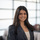# Confidence Interval Printout - R

Let's say we want a printout of our confidence interval for an entire sample (Note, if you're looking for a visual of a confidence interval over time, check out the post here!) The solution here requires Periscope Data's Python/R Integration as we'll be using R.

Notice that the function created in the R snippet takes in the following parameters for added customization:

• data: a vector that contains all of the values of a sample (in this example, we took a column from the built in cars dataframe. Of course, you can easily have this be a column of your SQL output)
• level: the width of the Confidence Interval. Default is set to 0.95 (95%)
• test_type: whether we use the t distribution ('T') or a z/normal distribution ('Z') to calculate the confidence interval. We recommend verifying that your data is normally distributed before using the z distribution statistic.
```# SQL output is imported as a dataframe variable called "df"
# Use Periscope to visualize a dataframe or show text by passing data to periscope.table() or periscope.text() respectively. Show an image by calling periscope.image() after your plot.

#As an exmaple, use the default Cars dataset and assign to df
df <- cars

confidence_interval <- function(data, level, test_type) {
ci_level <- (level + 1)/2.0
n <- length(data)
stdev <- sd(data)
mu <- mean(data)
if (test_type == 'T') {
error <- qt(ci_level,df=n-1)*stdev/sqrt(n)
}
else {
error <- qnorm(ci_level)*stdev/sqrt(n)
}
lower <- round(mu - error,2)
upper <- round(mu + error,2)
return(paste(paste(level * 100,'%',sep = ''),'Confidence Interval:',lower,'to',upper, sep = ' '))
}

periscope.text(confidence_interval(df\$dist, 0.95, 'Z'))
```

For the Python equivalent of this community post, check out the page here!

Found this useful? Let us know in the comments!